omG8HI9LSKFW6lhCj8prTs0Z6lUhdOT9Jhi1Sf4m

# Supernode Electric Circuits Analysis Easy Solving

Like we have learned in the previous post, the nodal analysis uses nodal voltages to analyze an electrical circuit. We also notice that we avoided using a voltage source in the circuit if we use this method.

Do you know why?

It is because a voltage source needs us to modify the nodal analysis for a little bit. But it doesn't mean we can ignore this method if there is a voltage source.

So what we can do actually? Let us change nodal analysis to supernode analysis. Even we need some modification, this method is easy even to nodal analysis.

I believe if you have understood the whole process of nodal analysis, this supernode analysis will be a piece of cake.

So let's get started.

Make sure to read what is dc circuit first.

## Supernode Analysis Theory

What is a supernode actually? Is it easier to use nodal analysis than supernode analysis? Well, you may be right, or not.

While nodal analysis is certainly easier, it is limited only to the current source. A mere nodal analysis will not be able to analyze an electrical circuit which has a voltage source.

For a start, just take a look at the circuit in Figure.(1). Can you see the difference between the circuit below with the circuits we have in the previous post about nodal analysis?

Congratulations if you have spotted the difference!

We don't have the current source but voltage sources only. What can we get from the circuit?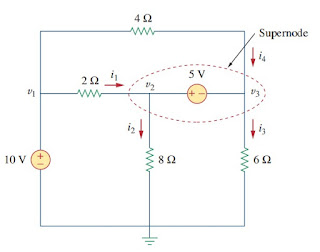Figure 1. Supernode electric circuit
The circuit above leads us to two similar possibilities :

CASE 1 - Take a look at the 10 V source on the most left branch. The voltage source is connected to the reference node (ground) and nonreference node (v1).

This condition simplifies our job to find the nodal voltages v1 because it has the same value as the voltage source connected to it in terms of CASE 1.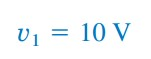(1)
Hence, our job in the analysis is simplified by this knowledge of voltage at this node. Let us move to the second case, it is harder to understand but deal with it.

CASE 2 - Unlike the first case, this one is when a voltage source is connected between two nonreference nodes (v2 and v3 ). These two nonreference nodes form a generalized node or supernode for short. We can use both KCL and KVL to calculate the node voltages.

Keep in mind :
A supernode is formed when a voltage source is connected between two nonreference nodes and any elements connected in parallel with it.
Like we learned about nodal analysis, we only need to use KCL to find the current flowing in each element. But when it comes to a supernode, it is impossible to calculate how much current is flowing through a voltage source.

What do we do then? Make sure to remember we need to satisfy KCL at a supernode like any other node.

Thus, at the supernode in Figure.(1),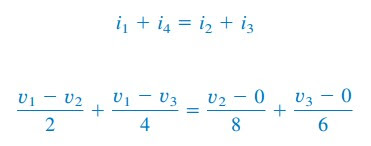(2)
We need to use KVL to the supernode in Figure.(1), but we redraw the circuit first to Figure.(2).Figure 2. Loop in the supernode
Make a loop in the clockwise direction gives :(3)
Since we have Equations.(1) and (2), obtaining the node voltages will be fairly easy.
Give more attention to these properties of a supernode :
1. The voltage source inside the supernode provides a constraint equation needed to solve for the node voltages.
2. A supernode has no voltage of its own.
3. A supernode requires the application of both KCL and KVL.

## Procedure for Nodal Analysis

We will call back what is the procedure for nodal analysis :
Identify all the nodes in the circuit
Set a node as a reference node. It usually acts as a ground so just add ground symbol to it.
Assign node voltage to other nodes (v1v2v3,etc)
Write all the KCL equations you can find (make the sum of the leaving current from a branch equal to zero)
Use substitution, elimination, Cramer's rule, etc.

For supernode analysis, the step will likely the same as the nodal analysis. To make it easier, just jump to examples below. Have fun!

## Supernode Analysis Examples

For better understanding let us review the examples below.
1. For the circuit shown in Figure.(3), find the node voltages.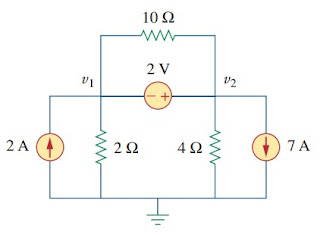Figure 3
Solution :
The supernode contains the 2 V source, nodes 1 and 2, and 10 Ω resistor.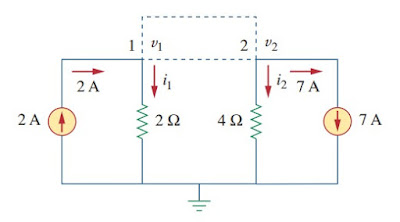Figure 4a
Applying KCL to the supernode in Figure.(4a) gives
Expressing i1 and i2 in terms of the node voltages,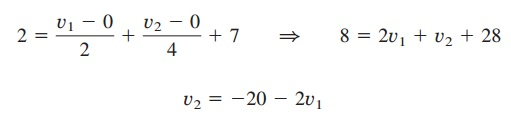(1.1)
To get the relationship between v1 and v2, we apply KVL to the circuit in Figure.(4b),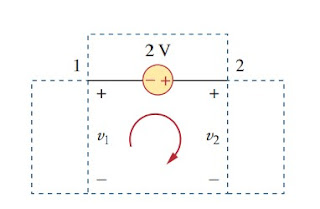Figure 4b
Going around the loop, we obtain
and v2 = v1 + 2 = -5.333 V. Note that the 10 Ω resistor does not make any difference because it is connected across the supernode.

2. Find the node voltages in the circuit of Figure.(5)
Nodes 1 and 2 form a supernode, also do nodes 3 and 4. We apply KCL to the two supernodes as in Figure.(6a).
Expressing this in terms of the node voltages,(2.2)
We now apply KVL to the branches involving the voltage sources as shown in Figure.(6b).(2.5)
We need four-node voltages, v1v2v3, and v4, and it requires only four out of the five (2.1) to (2.5) to find them. Although the fifth equation is redundant, it can be used to check results.
Substituting (2.3) to both (2.1) and (2.2) respectively gives(2.7)
(2.4) , (2.6) and (2.7) can be replaced in matrix form as

Have you understood what is supernode analysis? Don't forget to share and subscribe! Happy learning!
Reference:  Fundamentals of electric circuits by Charles K. Alexander and Matthew N. O. Sadiku

Untuk Bahasa Indonesia baca Analisis Supernode.
Related Posts
SHARE